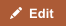Heat and temperature are related and often confused. More heat usually means a higher temperature.

Heat (symbol: Q) is energy. It is the total amount of energy (both kinetic and potential) possessed by the molecules in a piece of matter. Heat is measured in Joules.

Temperature (symbol: T) is not energy. It relates to the average (kinetic) energy of microscopic motions of a single particle in the system per degree of freedom. It is measured in Kelvin (K), Celsius (C) or Fahrenheit (F).

When you heat a substance, either of two things can happen: the temperature of the substance can rise or the state of substance can change.

## Comparison chart

Heat versus Temperature comparison chartHeatTemperature
Definition Heat is energy that is transferred from one body to another as the result of a difference in temperature. Temperature is a measure of hotness or coldness expressed in terms of any of several arbitrary scales like Celsius and Fahrenheit.
Unit Joules Kelvin, Celsius or Fahrenheit
Symbol Q T
SI unit Joule Kelvin
Particles Heat is a measure of how many atoms there are in a substance multiplied by how much energy each atom possesses. Temperature is related to how fast the atoms within a substance are moving. The ‘temperature’ of an object is like the water level – it determines the direction in which ‘heat’ will flow.
Ability to do work Heat has the ability to do work. Temperature can only be used to measure the degree of heat.

## Relationship Between Heat and Temperature

In the video below, Derek Muller of Veritasium goes to the streets to show strangers how two items may have the same temperature but conduct heat differently, therefore feeling either warmer or cooler to the touch.